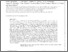# The nature of sub-millimeter and highly star-forming galaxies in the EAGLE simulation

McAlpine, S, Smail, I, Bower, RG, Swinbank, MA, Trayford, JW, Theuns, T, Baes, M, Camps, P, Crain, RA and Schaye, J (2019) The nature of sub-millimeter and highly star-forming galaxies in the EAGLE simulation. Monthly Notices of the Royal Astronomical Society, 488 (2). pp. 2440-2454. ISSN 0035-8711Preview
Text
mcalpine.pdf - Accepted Version

We exploit EAGLE, a cosmological hydrodynamical simulation, to reproduce the selection of the observed sub-millimeter (submm) galaxy population by selecting the model galaxies at $z \geq 1$ with mock submm fluxes $S_{850} \geq 1$ mJy. There is a reasonable agreement between the galaxies within this sample and the properties of the observed submm population, such as their star formation rates (SFRs) at $z<3$, redshift distribution and many integrated galaxy properties. We find that the bulk of the $S_{850} \geq 1$ mJy model population is at $z = 2.5$, and that they are massive galaxies ($M_* \sim 10^{11}$ Msol) with high dust masses ($M_{\mathrm{dust}} \sim 10^{8}$ Msol), gas fractions ($f_{\mathrm{gas}} \approx 50$%) and SFRs ($\dot M_* \approx 100$ Msol/yr). They have major and minor merger fractions similar to the general population, suggesting that mergers are not the primary driver of the model submm galaxies. Instead, the $S_{850} \geq 1$ mJy model galaxies yield high SFRs primarily because they maintain a significant gas reservoir as a result of hosting an undermassive black hole. In addition, we find that not all highly star-forming EAGLE galaxies have submm fluxes $S_{850} > 1$ mJy. Thus, we investigate the nature of $z \geq 1$ highly star-forming Submm-Faint galaxies (i.e., $\dot M_* \geq 80$ Msol/yr but $S_{850}< 1$ mJy). We find they are similar to the model submm galaxies; being gas rich and hosting undermassive black holes, however they are typically lower mass ($M_* \sim 10^{10}$ Msol) and are at higher redshifts ($z>4$). These typically higher-$z$ galaxies show stronger evidence for having been triggered by major mergers, and critically, they are likely missed by current submm surveys due to their higher dust temperatures. This suggests a potentially even larger contribution to the SFR density at $z > 3$ from dust-obscured systems than implied by current observations.View Item